# Consumer Equilibrium – Income Effect and Income Consumption CurveIncome Consumption Curve refers to the effect of the change in income on the consumer equilibrium. Consumer equilibrium is affected by the change in income, change in price and change in the prices of substitute goods. In this article, we will discuss the effect of change in income on the equilibrium of the consumer.

## Income Effect :

The income effect may be defined as the effect on the purchases of the consumer due to change in the income, provided the prices being the same. It implies, that the increase in income increases the satisfaction and the equilibrium point shifts upward to the right. Similarly, the fall in income decreases the satisfaction and equilibrium point shifts downwards to the left.

### Graphical Representation:

The income effect can be understood with the following graphical representation: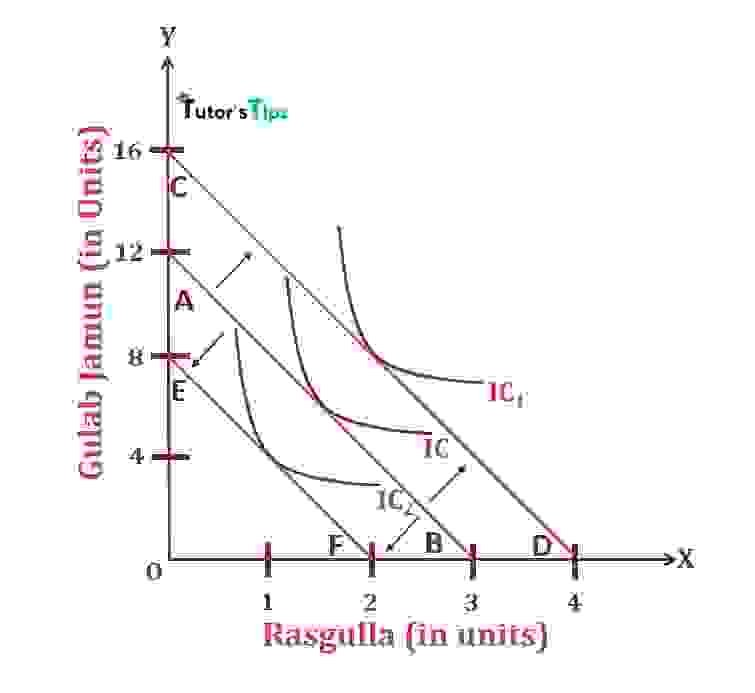Consumer Equilibrium-Income effect

In the above fig, AB is the original budget line and IC is the main indifference curve. Here, the point E refers to the consumer equilibrium where the consumer buys 3 units of rasgulla and 12 units of Gulab Jamun. When the income of consumer increases, it enables him to buy more quantity of both commodities at given prices. In other words, when the income of the consumer increases then the budget line will shift to the right as shown by budget line CD.

Similarly, when income decreases, it enables the consumer to buy fewer units of both commodities. Consequently, the budget line shifts downwards as shown by budget line EF. However, it is assumed that the prices of both commodities remain unchanged i.e. PX/PY remain constant. Consequently, the slope of all budget lines also remains the same. In other words, when there is a change in income, the budget lines remain parallel to each other.

## Income Consumption Curve:

It is the curve that shows equilibrium quantities of two commodities that would be purchased by the buyer at different levels of income, keeping prices the same.

In the words of Ferguson,

” The income consumption curve is the curve which shows the points of equilibrium resulting  from various levels of money income and constant prices.”

### Explanation with Graphical Representation:

The income consumption curve is explained with the following graphical representation: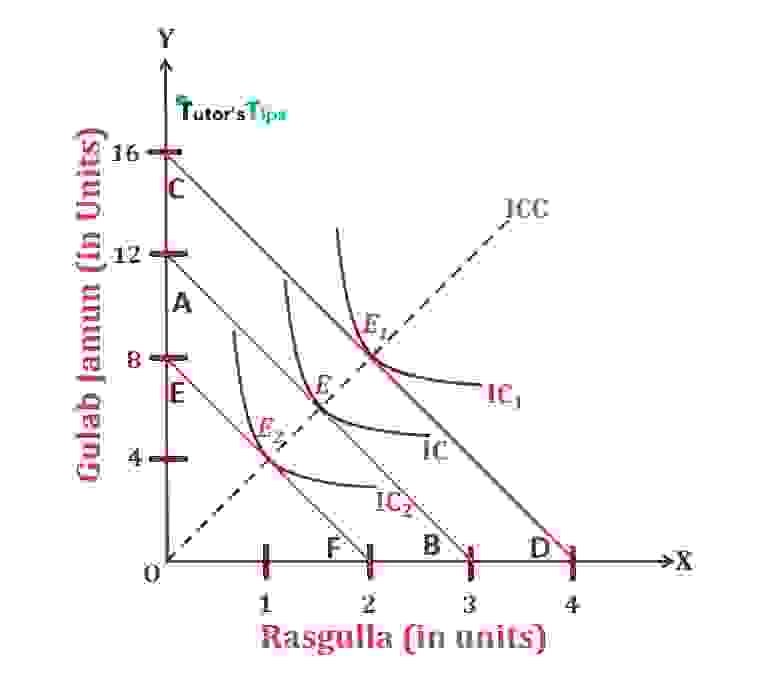Income consumption curve

In fig, X-axis shows the quantity of rasgulla and Y-axis shows the quantity of Gulab Jamun. The income is shown by budget line AB and E is the equilibrium point where the budget line is tangent to an indifference curve. When the income of consumer increases, the equilibrium point and budget line shifts to the right i.e. E1 on the budget line CD. Similarly, with a fall in the income, the consumer’s equilibrium and price or budget line shift to E2 on EF. Thus,  the line joining points E, Eand E2, is called Income Consumption Curve. Thus, ICC shows the quantities of rasgulla and Gulab Jamun, the consumer buys at different levels of income.

### The slope of the Income Consumption Curve :

The slope of the ICC curve varies with the type of goods involved. It can be classified as :

1. Positive Sloped ICC curve
2. Negative Sloped ICC curve

These can be explained as:

#### Positive Sloped Income Consumption Curve :

The slope of ICC is positive in case of normal goods.  As the consumption of both normal goods increases with the increase in income, the positive relation is defined. Hence, it is positively sloped if both goods are normal.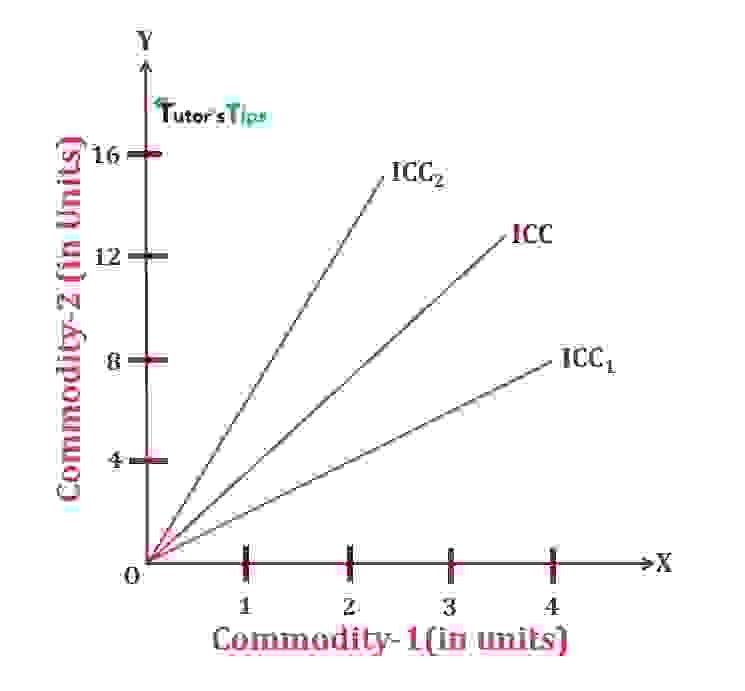Positive sloped ICC

In the above fig, it is shown that ICC of normal goods slopes upwards from left to right indicating that there will be an increase in the consumption of both goods with an increase in income. In fig, ICC curve implies that the same proportionate increase in the consumption of both commodities with a rise in income. ICC1 curve indicates the more proportionate increase in commodity-1 and ICCcurve indicates the more proportionate increase in commodity-2.

#### Negative Sloped Income Consumption Curve :

The slope of the ICC is negative in the case of inferior goods. It implies, that the consumption of inferior goods declines with the increase in income and the inverse relation is defined. Hence, it is negatively sloped if any or both of the goods are inferior goods.

##### Graphical Representation:
###### 1. When one commodity is inferior and another commodity is normal:

Suppose, commodity-1 is inferior and commodity-2 is normal. The following graph shows the relation of ICC when any of the commodity is inferior.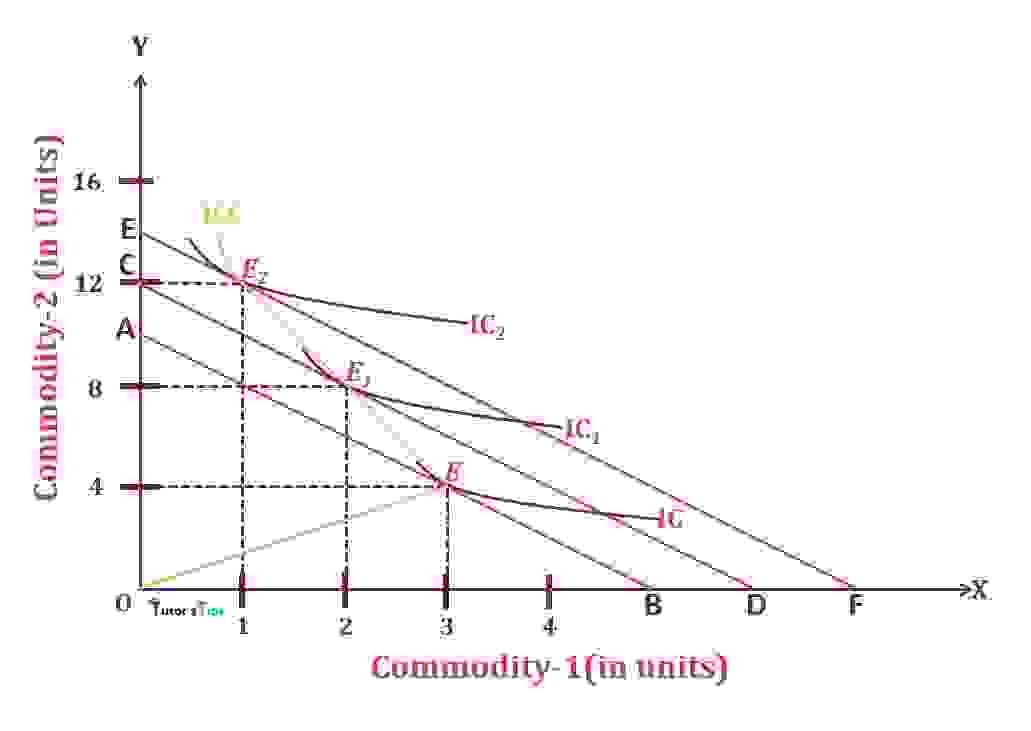Negative sloped ICC with one inferior commodity

In fig, X-axis shows the quantity of inferior commodity-1 and Y-axis shows the quantity of normal commodity-2. Here, AB is the price line, drawn based on the income of consumer and given prices of two commodities, touches the indifference curve IC at the point E(Equilibrium Point). As the income of consumer increases, the price line shifts to the right to CD and then EF touching IC1

and IC2 at the equilibrium points Eand E2 respectively. Consequently, the quantity of commodity-1 decreases from 3 units to 2 units and 1 unit respectively. Hence, the increase in income of the consumer is followed by a decrease in the demand for inferior commodity -1. The decreased income reflects the negative income effect.

By joining together different equilibrium points E, Eand E2, we get the consumption curve that slopes back to the left. It indicates the negative income effect. Also, from fig, it is evident that the demand for normal commodity-2 increases from 4 units to 8 units and then to 12 units as the income of consumer increases. Thus, the income effect on normal goods is positive.

###### 2.When both commodities are inferior goods.

Suppose, both the commodities which are purchased by the consumer are inferior goods. The following graph shows the relation of ICC when both commodities are inferior.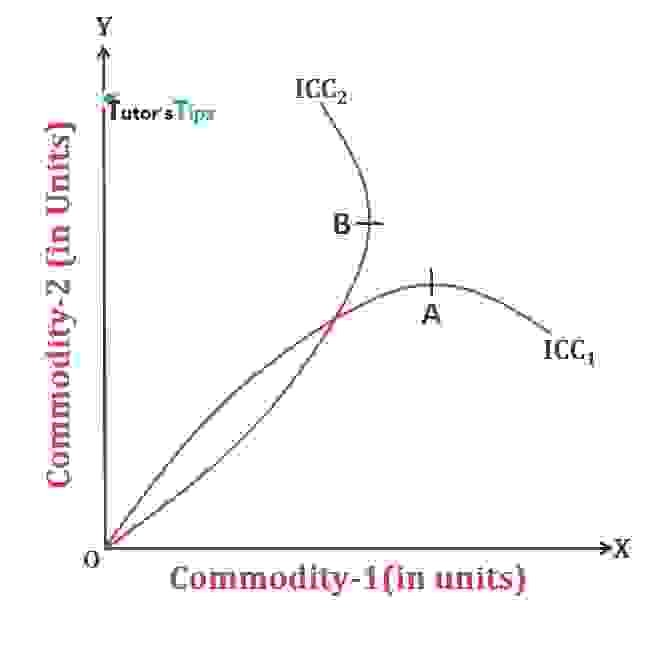Negative sloped ICC with inferior commodities

In fig, ICCcurve shows that commodity-2 is an inferior commodity. This curve turns downwards after point A. It means that less of the commodity-2 will be purchased when the income of consumer increases. Similarly, ICCcurve shows the inferior commodity-1. This curve turns backwards after point B which means that less of commodity-1 will be purchased when the income of consumer increases.

Thanks!!

Please share with your friends

Comment if you have any question.

References:

Introductory Microeconomics – Class 11 – CBSE (2020-21)